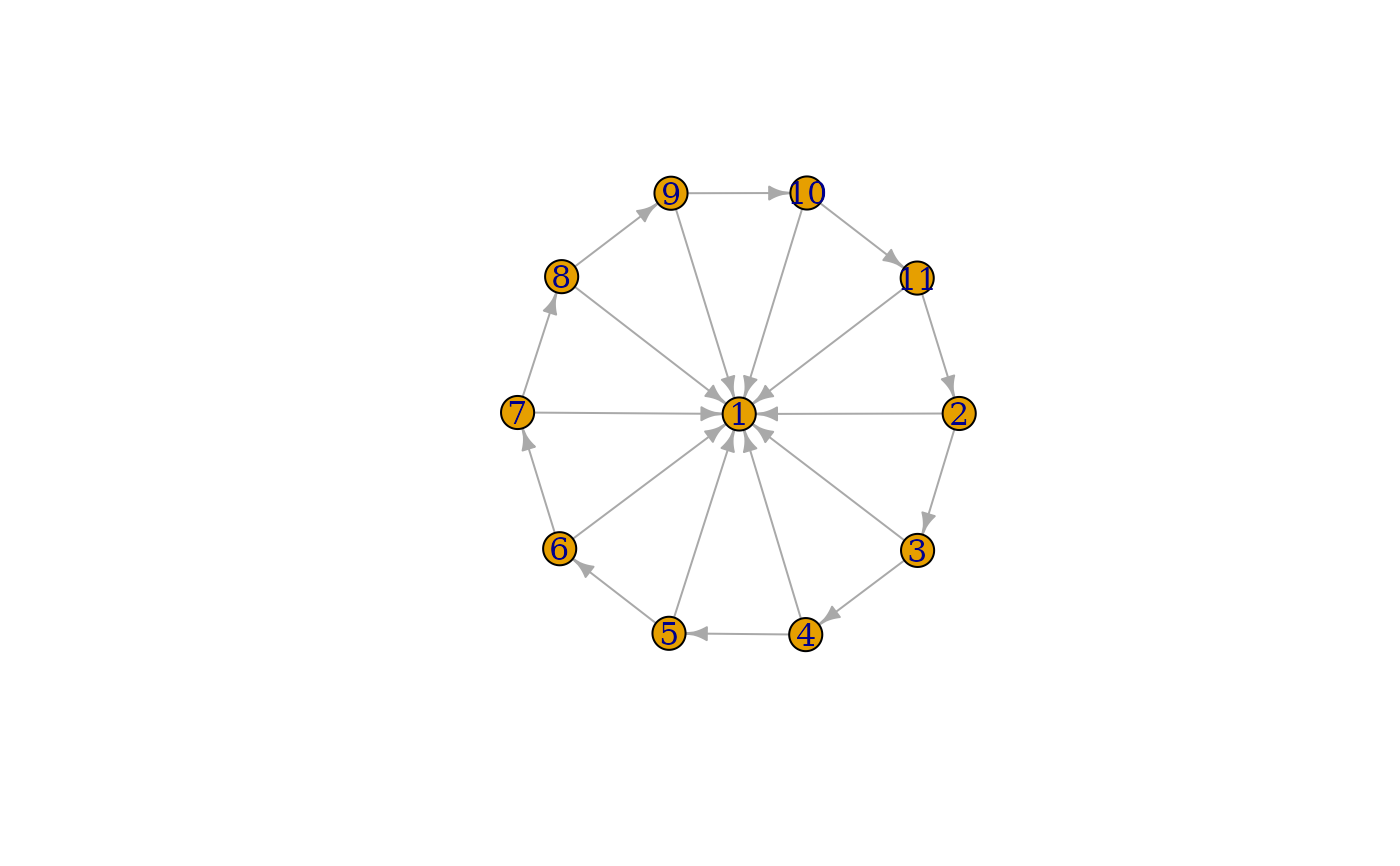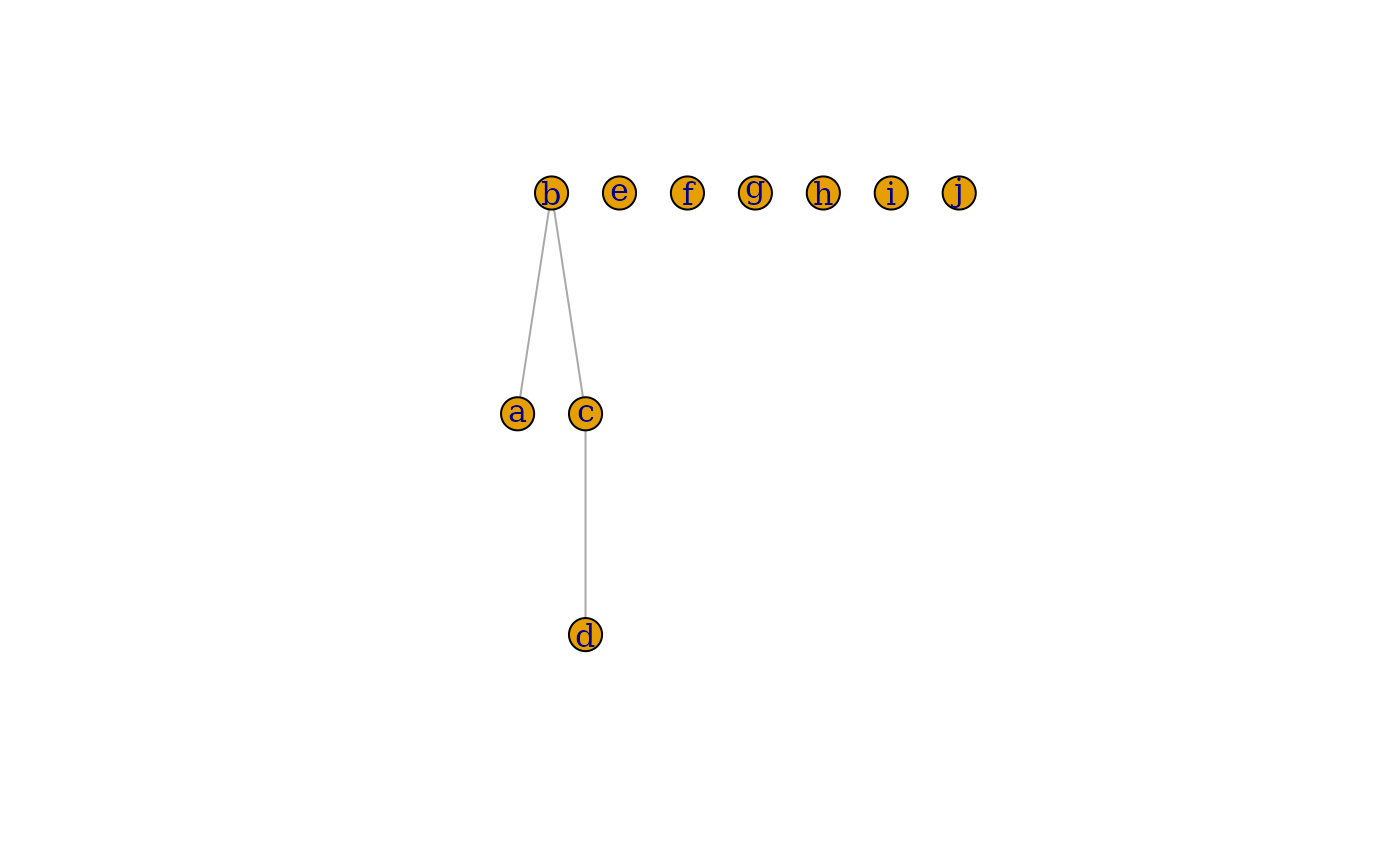This function can be used to add or delete edges that form a path.

## Usage

path(...)

## Arguments

...

See details below.

## Value

A special object that can be used together with igraph graphs and the plus and minus operators.

## Details

When adding edges via +, all unnamed arguments are concatenated, and each element of a final vector is interpreted as a vertex in the graph. For a vector of length $$n+1$$, $$n$$ edges are then added, from vertex 1 to vertex 2, from vertex 2 to vertex 3, etc. Named arguments will be used as edge attributes for the new edges.

When deleting edges, all attributes are concatenated and then passed to delete_edges().

Other functions for manipulating graph structure: +.igraph(), add_edges(), add_vertices(), complementer(), compose(), connect(), contract(), delete_edges(), delete_vertices(), difference.igraph(), difference(), disjoint_union(), edge(), igraph-minus, intersection.igraph(), intersection(), permute(), rep.igraph(), reverse_edges(), simplify(), union.igraph(), union(), vertex()

## Examples

# Create a (directed) wheel
g <- make_star(11, center = 1) + path(2:11, 2)
plot(g)g <- make_empty_graph(directed = FALSE, n = 10) %>%
set_vertex_attr("name", value = letters[1:10])

g2 <- g + path("a", "b", "c", "d")
plot(g2)g3 <- g2 + path("e", "f", "g", weight = 1:2, color = "red")
E(g3)[[]]
#> + 5/5 edges from 89ef06f (vertex names):
#>   tail head tid hid weight color
#> 1    a    b   1   2     NA  <NA>
#> 2    b    c   2   3     NA  <NA>
#> 3    c    d   3   4     NA  <NA>
#> 4    e    f   5   6      1   red
#> 5    f    g   6   7      2   red

g4 <- g3 + path(c("f", "c", "j", "d"), width = 1:3, color = "green")
E(g4)[[]]
#> + 8/8 edges from a304794 (vertex names):
#>   tail head tid hid weight color width
#> 1    a    b   1   2     NA  <NA>    NA
#> 2    b    c   2   3     NA  <NA>    NA
#> 3    c    d   3   4     NA  <NA>    NA
#> 4    e    f   5   6      1   red    NA
#> 5    f    g   6   7      2   red    NA
#> 6    c    f   3   6     NA green     1
#> 7    c    j   3  10     NA green     2
#> 8    d    j   4  10     NA green     3